## 26 Dec 2018

### Wildberger (31) WildTrig: Intro to Rational Trigonometry, 31: “Introduction to Projective Geometry, summary

[Search Blog Here. Index tabs are found at the bottom of the left column.]

[Central Entry Directory]

[Mathematics, Calculus, Geometry, Entry Directory]

[Norman Wildberger, entry directory]

[Wildberger, Wildtrig, entry directory]

[The following is summary of Wildberger’s video lecture. You will find that he is a supremely talented teacher. Any mistakes are my own, as I am not a mathematician. Geometry graphics not from the video are made using Geogebra.]

Norman J. Wildberger

Course Series

WildTrig: Introduction to Rational Trigonometry

31

“Introduction to Projective Geometry”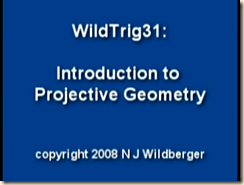(Image source: Wildberger)

Brief summary:

(31.1) Wildberger introduces the importance of projective geometry. (31.2) Pappus showed the following, which is called “Pappus’ Theorem”. Take two lines and assign three points on each. Next find the intersection between: {a} the line formed from the 1st point on one line and the 2nd on the other with {b} the line formed from the 2nd point on the first line and the 1st on the other. Then make the other two unique such pairings. All three points of intersection will be co-linear (will lie on a shared line).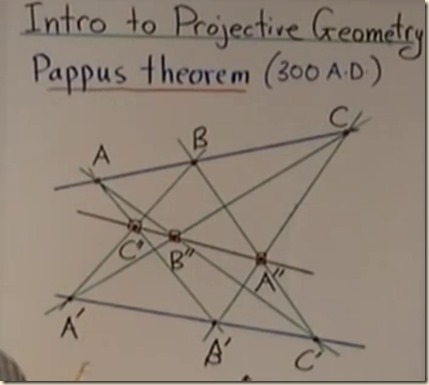(Image source: Wildberger)

(31.3) Suppose the two lines of Pappus’ Theorem are made to intersect and the point ordering is changed to C, B, A and C′, A′, B′. When the AB lines are parallel and when the AC lines are parallel, we can infer the following two things from the theorem. {1} The BC lines will be parallel. And {2} the parallel lines still somehow intersect (infinitely) far off at their pre-given point of intersection. In other words, this special case of the theorem gives us an instance where parallel lines intersect at infinity.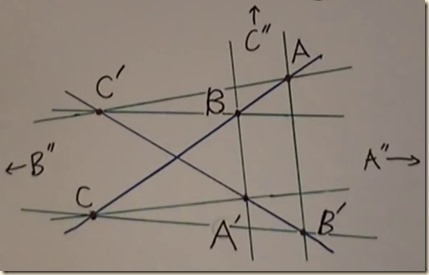(Image source: Wildberger)

(31.4) Desargues’ theorem says that if two triangles, ABC and A′B′C′ have vertices that are perspective from a point, then they are perspective from a line.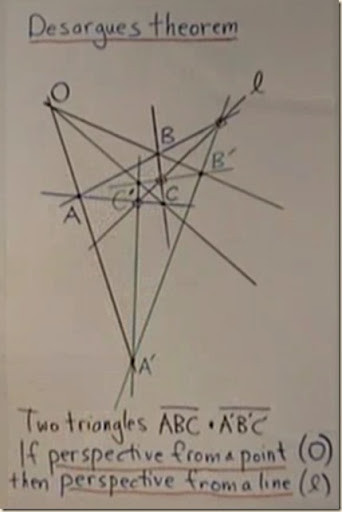(Image source: Wildberger)

(31.5) A quadrangle’s  or quadrilateral’s opposite can be formed in the following way. We begin with the four vertices of a quadrangle. We then draw lines through every pairing, giving us six. These lines have their own new points of intersection between them. There are three such points of intersection, called the diagonal points of the quadrangle, and the triangle they form is called the diagonal triangle of the original quadrangle. The lines of this triangle intersect the lines of the quadrangle in six locations. Those six points can be grouped into four co-linear triples. The lines made by those co-linear triples form a new quadrilateral, which is the opposite quadrilateral to the original quadrangle.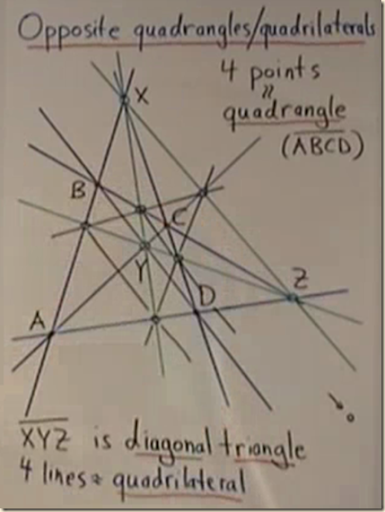(Image source: Wildberger)

Contents

31.1

Introductory Material

31.2

Pappus’ Theorem, Normal Case

31.3

Pappus’ Theorem, Special Case

31.4

Desargues’ Theorem

31.5

Opposite Quadrangle/Quadrilateral (Theorem)

Summary

31.1

Introductory Material

(00.05-00.23)

[Wildberger introduces the importance of projective geometry.]

[ditto]

[contents]

31.2

Pappus’ Theorem, Normal Case

(00.24-01.31)

[Pappus showed the following, which is called “Pappus’ Theorem”. Take two lines and assign three points on each. Next find the intersection between: {a} the line formed from the 1st point on one line and the 2nd on the other with {b} the line formed from the 2nd point on the first line and the 1st on the other. Then make the other two possible pairings as such. All three new points of intersection will be co-linear (will lie on a shared line).(Image source: Wildberger)]

[The subject of projective geometry goes back to the ancient Greeks, namely, to Pappus. We will now examine Pappus theorem (300 AD). We start with two lines.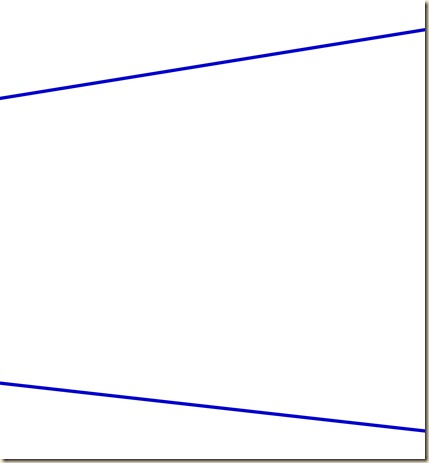We then choose on the first line three points, A, B, C, and on the other line, A′, B′, C′.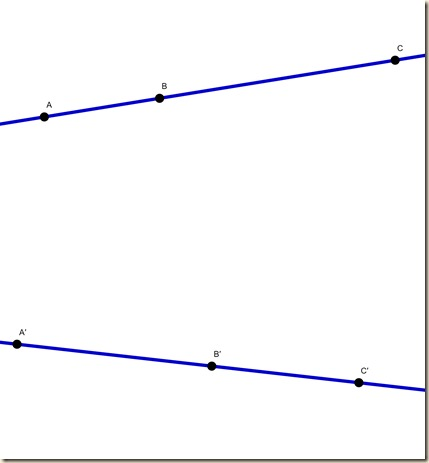We next connect A to B′ with a line.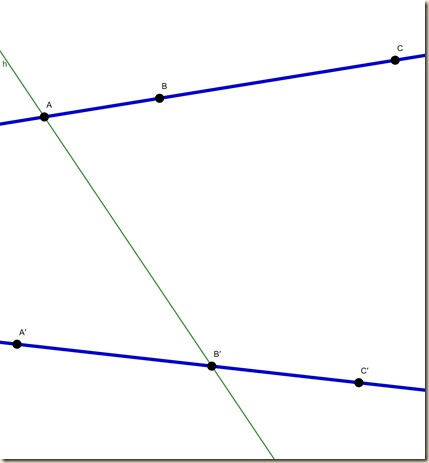Then B to A′ with a line.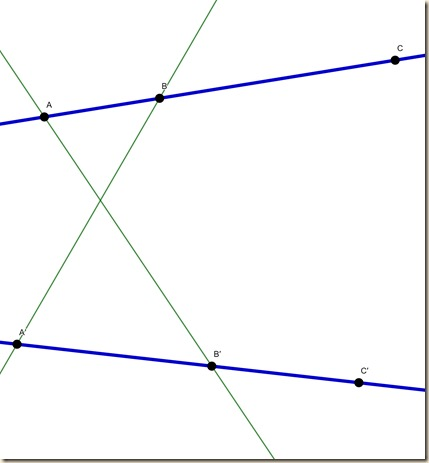And we call the point of that intersection, C″.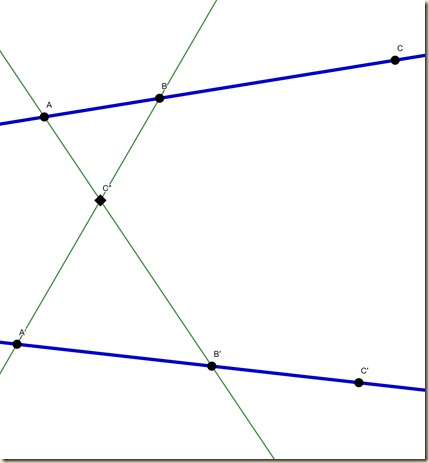We do the same for A to Cand C to A′, and we call that point of intersection B. ]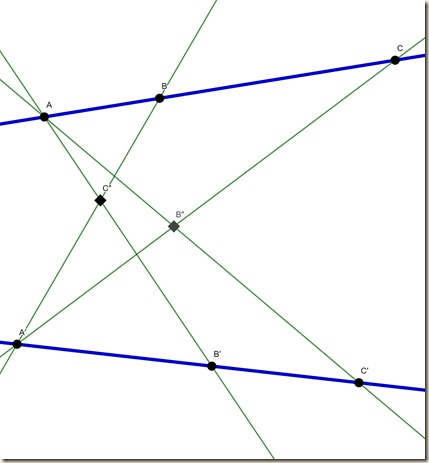And we then do the same for B to Cand C to B, and we call that point of intersection A.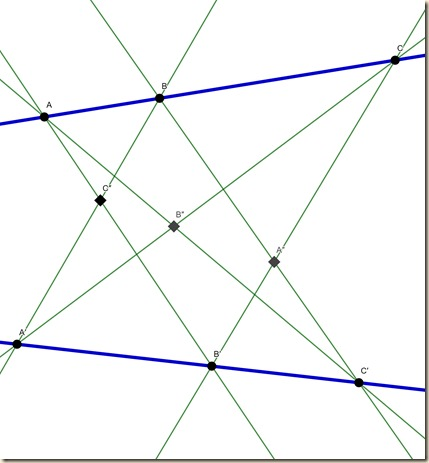Pappus thereby showed that these three points of intersection are collinear (they lie on a common line).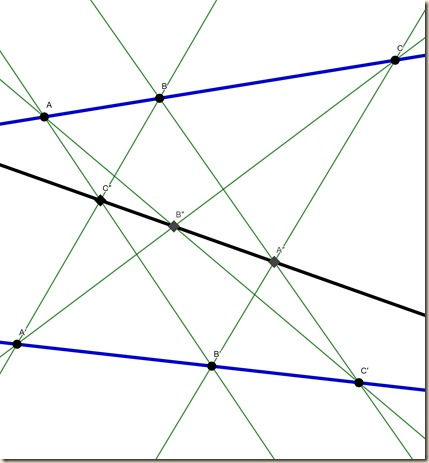Here is Wildberger’s drawing:(Image source: Wildberger)]

[contents]

31.3

Pappus’ Theorem, Special Case

(01.31-02.14)

[Suppose the two lines of Pappus’ Theorem are made to intersect and the point ordering is changed to C, B, A and C′, A′, B′. When the AB lines are parallel and when the AC lines are parallel, we can infer the following two things from the theorem. {1} The BC lines will be parallel. And {2} the parallel lines still somehow intersect (infinitely) far off at their pre-given point of intersection. In other words, this special case of the theorem gives us an instance where parallel lines intersect at infinity.(Image source: Wildberger)]

[We next consider a special case of this demonstration. We will rearrange the points and lines into the following configuration.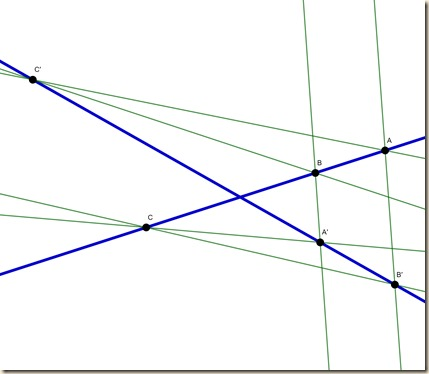(Image source: Wildberger)

Here the AB lines and the CA lines are parallel. When they are parallel, the intersections no longer exist; nonetheless, Pappus’ theorem, Wildberger says, still has some “content.” For instance, the theorem says that the BC lines might also be parallel. (But, under our suppositions, these parallel lines share a point of intersection. Thus), “somehow parallel lines meet at infinity in some nebulous way.” (Note, consider the following movements, firstly of the AB lines toward parallel, then at parallel, and then moving away from parallel, and likewise for the AC lines.

For the AB lines, their point of intersection C″ starts above the figure, then after the parallelism, it appears at the bottom. Perhaps at parallel they leave the finite scale. Something equivalent happens for the A″ and B″ points when the AC and BC lines become parallel, respectively. So how can these intersection points be on the same line but be off infinitely in differing directions?(Image source: Wildberger)

The only thing that comes to my imagination is something like the line is above them on something like a third dimension, and the arrows here are pointing upward. Perhaps we will learn what is going on later.)]

[contents]

31.4

Desargues’ Theorem

(02.14-04.10)

[Desargues’ theorem says that if two triangles, ABC and A′B′C′ have vertices that are perspective from a point, then they are perspective from a line.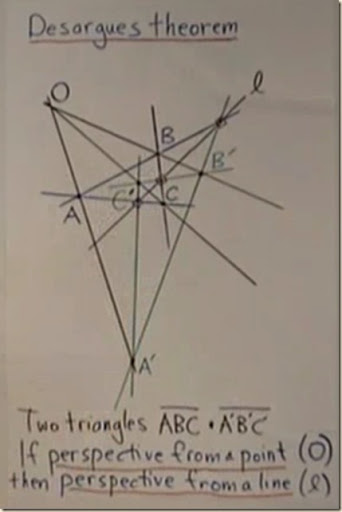(Image source: Wildberger)]

[We turn now to Desargues’ theorem. Desargues is perhaps the founder of modern projective geometry.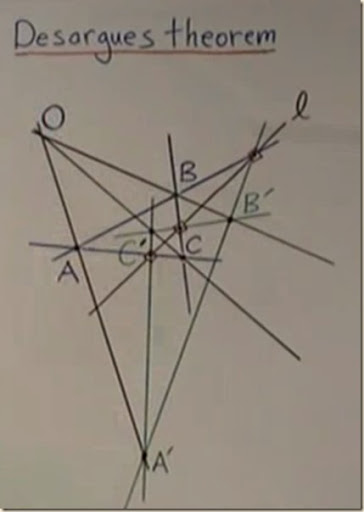(Image source: Wildberger)

We begin with a triangle whose three points, A, B, and C are “perspective” from a point O.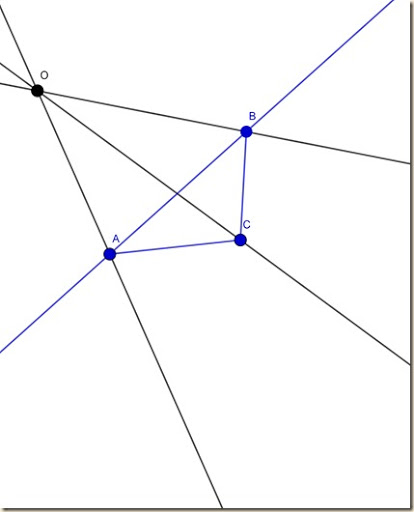Then we take another triangle whose points A′, B′, and C′ are also perspective from the same point O.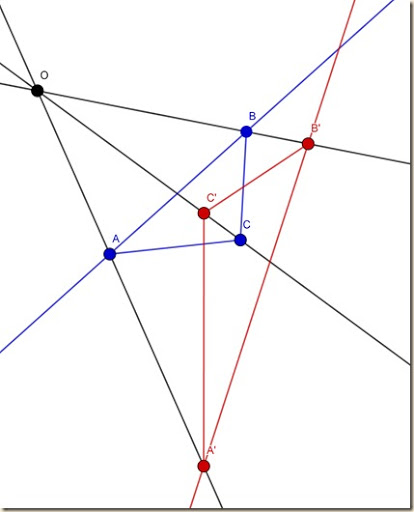We next look at the AB sides on both triangles.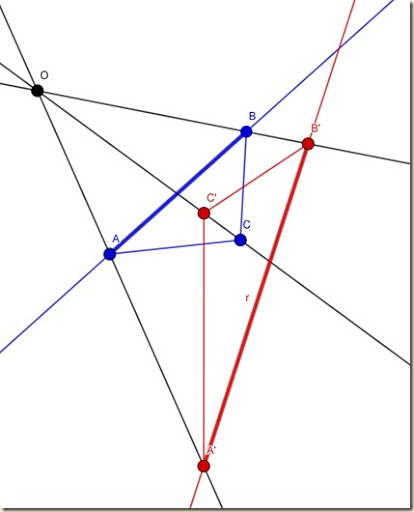We see that their lines (when extended) intersect at a particular point.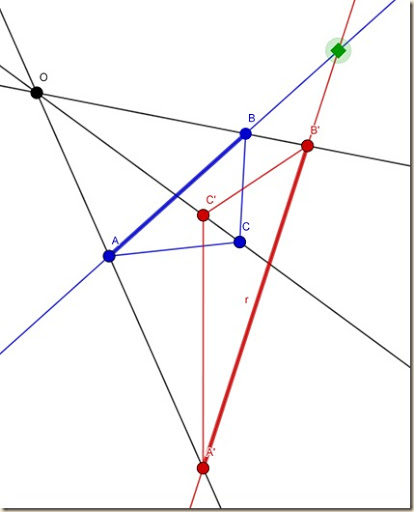We can next find the intersection of the BC sides.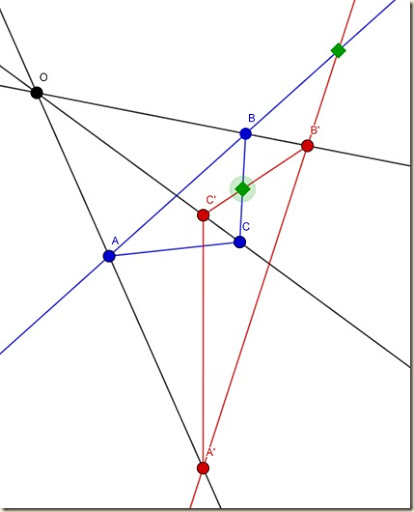And again for the AC sides.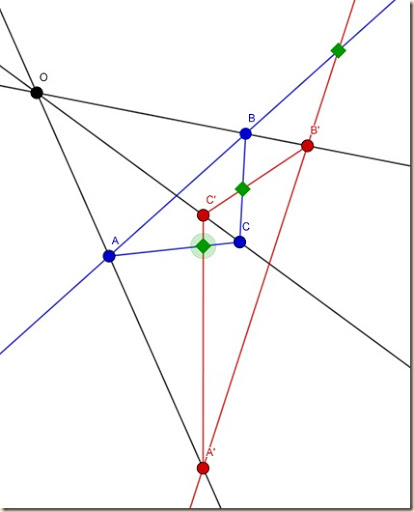As we can see, these three points fall upon a line, ℓ.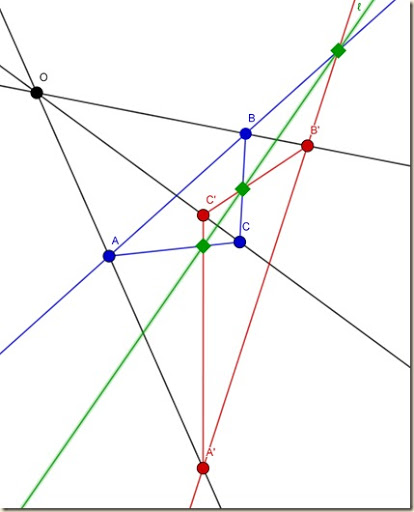This means that the two triangles (or the two sets of vertices) are “perspective from a line”. (This is harder for me to understand than being perspective from a point. It seems to mean one single straight line intersects the points of intersection of the respective triangle sides. But I do not have an intuition for the notion like I have for a perspective from a point.) Desargues’ theorem says that if two triangles, ABC and A′B′C′ have vertices that are perspective from a point, then they are perspective from a line.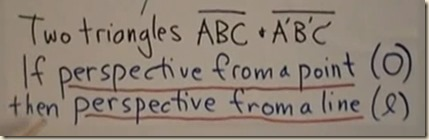(Image source: Wildberger)

[contents]

31.5

Opposite Quadrangle/Quadrilateral (Theorem)

(04.11-end)

[A quadrangle’s or quadrilateral’s opposite can be formed in the following way. We begin with the four vertices of a quadrangle. We then draw lines through every pairing, giving us six. These lines have their own new points of intersection between them. There are three such points of intersection, called the diagonal points of the quadrangle, and the triangle they form is called the diagonal triangle of the original quadrangle. The lines of this triangle intersect the lines of the quadrangle in six locations. Those six points can be grouped into four co-linear triples. The lines made by those co-linear triples form a new quadrilateral, which is the opposite quadrilateral to the original quadrangle.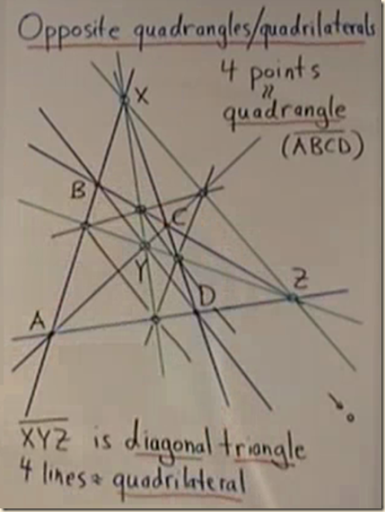(Image source: Wildberger)]

[We will now examine the opposite quadrangles/quadrilaterals. A quadrangle is a four-angled figure, and a quadrilateral is a four-sided figure (they form the same figure, but the sides of the quadrilateral will extend outside the enclosed tetragon figure.) This theorem will express the relation between the quadrangle and quadrilateral. (But it is still not entirely clear to me how they are differentiated and then related.) We first say that points ABCD form a quadrangle, and the four lines from one to the next form a quadrilateral. (And we are supposing that no three points are co-linear.)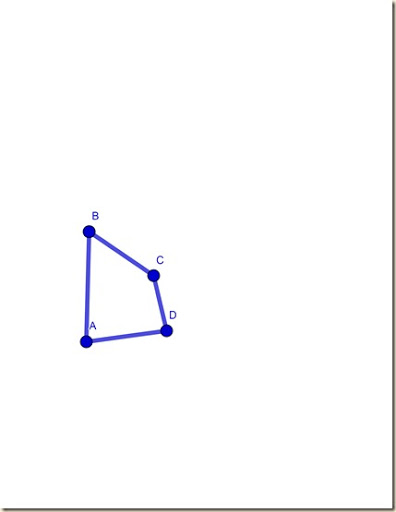To these points we can form six lines by connecting any two.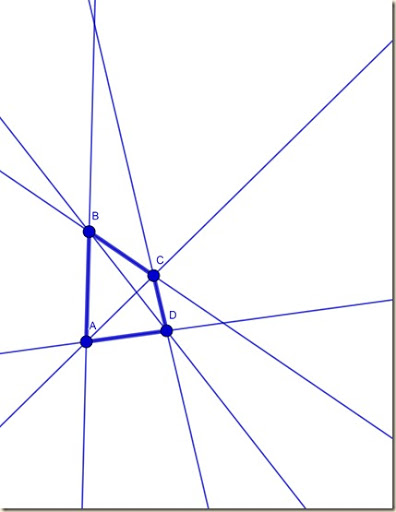These lines intersect at three additional points, X, Y, and Z, which are called the diagonal points of the quadrangle (shown in green below).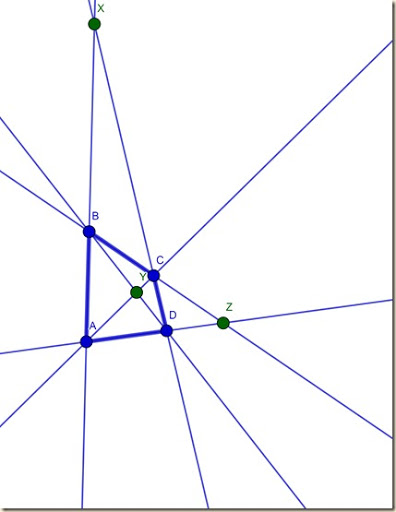We then draw the lines through those diagonal points (shown in green below).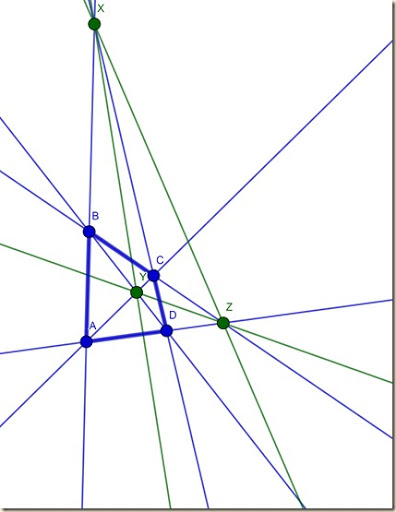These new lines then form a triangle with X, Y, and Z, and it is called the diagonal triangle of the original quadrangle (shown in bolded green below).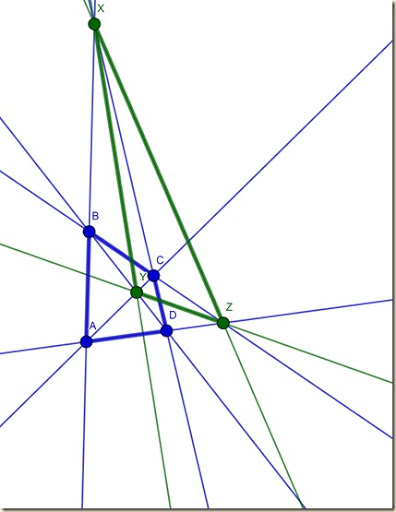Now, the lines of the triangle intersect the lines of the quadrangle in six locations (shown in red below).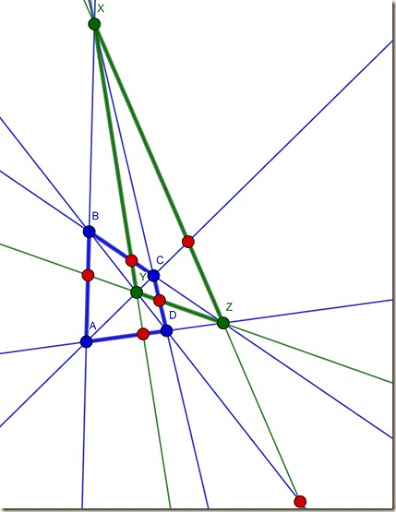These new six points form four new lines on the basis of their co-linearities (shown in red below).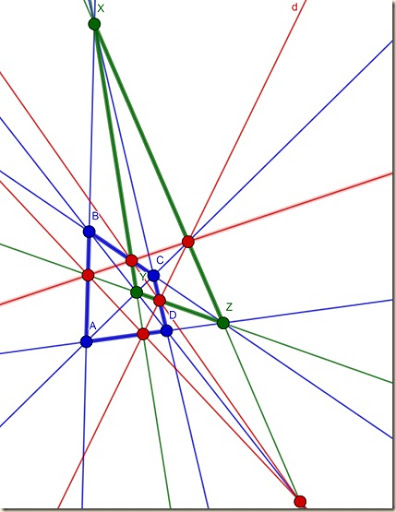This gives us four new lines forming a new quadrilateral, which is called the “opposite” quadrilateral to the quadrangle we started with (shown in bolded red below).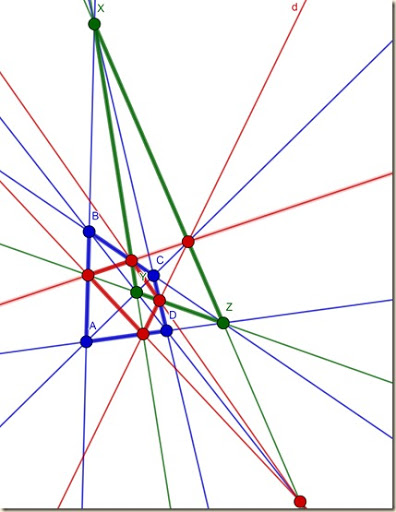]

[contents]

Wildberger, Norman J. (2008) WildTrig: Introduction to Rational Trigonometry, 31: “Introduction to Projective Geometry”. Course Series.

Youtube page:

https://youtu.be/fX7IOxuIjKY

Course Youtube Playlist:

https://www.youtube.com/watch?v=GGj399xIssQ&list=PL3C58498718451C47

Norman J. Wildberger, youtube channel:

njwildberger

https://www.youtube.com/channel/UCXl0Zbk8_rvjyLwAR-Xh9pQ